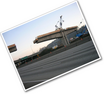Unit 4 Expressions

# Unit Description

A start at Algebra! Want to learn a math trick? Or how about build your own train? In this unit you will be exploring numerical and algebraic expressions. You will learn how to write and evaluate expressions while doing math tricks and modeling trains! Learn how to identify equivalent expressions and apply properties of operations, such as the distributive property. Finally, you will use geometric models to explore greatest common factors and least common multiples of number pairs.

•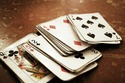Lesson 1

Math Tricks`
••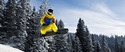•••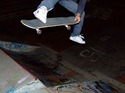•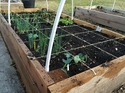••••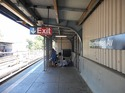Lesson 11

Peer Review`
•